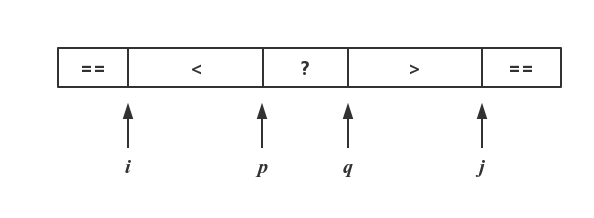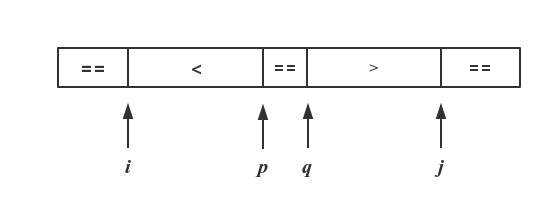快速排序不快了？重复元素下的快速排序• 快速排序的工作原理
• 重复元素下的快排
• 基本有序下数组下的快排

一、快速排序是如何工作的？

1.1.分区

arr[0...x-1] ≤ arr[x] ≤ arr[x+1...arr.length-1]

1.2.递归

public void quickSort(int[] arr, int low, int high) {
if (low < high) {
int pivot = partition(arr, low, high);
quickSort(arr, low, pivot - 1);
quickSort(arr, pivot + 1, high);
}
}

二、重复元素下的快速排序• 左右指针没有相遇前，pivotValue的最终位置无法确定
• 分区函数的目的是确定pivotValue最终的位置

while (p < q) {
while (p < q && arr[q] >= pivotValue) {
if (arr[q] == pivotValue) {
swap(arr, j, q);
j--;
}
q--;
}
while (p < q && arr[p] <= pivotValue) {
if (arr[p] == pivotValue) {
swap(arr, i, p);
i++;
}
p++;
}
swap(arr, p, q);
}

if (arr[p] == pivotValue) {
p--;
q++;
}• i，p指针所指向的位置交换，同时一起递减，直至i ≥ low
• j，q指针所指向的位置交换，同时一起递增，直至j ≤ high

while (i > low) {
swap(arr, i - 1, p);
p--;
i--;
}
while (j < high) {
swap(arr, j + 1, q);
q++;
j++;
}

private int[] partition3(int[] arr, int low, int high) {
int i = low;
int j = high;
int p = low;
int q = high;
int pivotValue = arr[low];
while (p < q) {
while (p < q && arr[q] >= pivotValue) {
if (arr[q] == pivotValue) {
swap(arr, j, q);
j--;
}
q--;
}
while (p < q && arr[p] <= pivotValue) {
if (arr[p] == pivotValue) {
swap(arr, i, p);
i++;
}
p++;
}
swap(arr, p, q);
}
if (arr[p] == pivotValue) {
p--;
q++;
}
while (i > low) {
swap(arr, i - 1, p);
p--;
i--;
}
while (j < high) {
swap(arr, j + 1, q);
q++;
j++;
}
return new int[]{p + 1, q - 1};
}

三、基本有序下数组下的快排

public void insertSort(int[] arr) {
int i;
int j;
for (i = 1; i < arr.length; i++) {
if (arr[i - 1] > arr[i]) {
int t = arr[i];
arr[i] = arr[i - 1];
for (j = i - 1; t < arr[j]; j--) {
arr[j + 1] = arr[j];
}
arr[j + 1] = t;
}
}
}